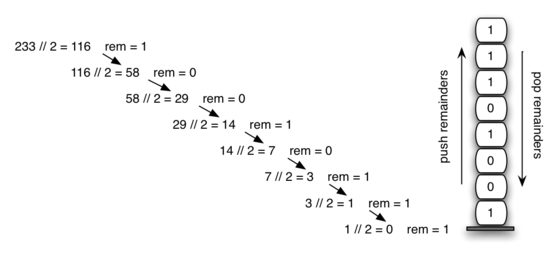# 3.8. Converting Decimal Numbers to Binary Numbers¶

In your study of computer science, you have probably been exposed in one way or another to the idea of a binary number. Binary representation is important in computer science since all values stored within a computer exist as a string of binary digits, a string of 0s and 1s. Without the ability to convert back and forth between common representations and binary numbers, we would need to interact with computers in very awkward ways.

Integer values are common data items. They are used in computer programs and computation all the time. We learn about them in math class and of course represent them using the decimal number system, or base 10. The decimal number $$233_{10}$$ and its corresponding binary equivalent $$11101001_{2}$$ are interpreted respectively as

$$2\times10^{2} + 3\times10^{1} + 3\times10^{0}$$

and

$$1\times2^{7} + 1\times2^{6} + 1\times2^{5} + 0\times2^{4} + 1\times2^{3} + 0\times2^{2} + 0\times2^{1} + 1\times2^{0}$$

But how can we easily convert integer values into binary numbers? The answer is an algorithm called “Divide by 2” that uses a stack to keep track of the digits for the binary result.

The Divide by 2 algorithm assumes that we start with an integer greater than 0. A simple iteration then continually uses integer division to divide the decimal number by 2 and to keep track of the remainder. The first division by 2 gives information as to whether the value is even or odd. An even value will have a remainder of 0. It will have the digit 0 in the 1s place. An odd value will have a remainder of 1 and will have the digit 1 in the 1s place. We think about building our binary number as a sequence of digits; the first remainder we compute will actually be the last digit in the sequence. As shown in Figure 5, we again see the reversal property that signals that a stack is likely to be the appropriate data structure for solving the problem.Figure 5: Decimal-to-Binary Conversion

The code in ActiveCode 1 implements the Divide by 2 algorithm. The function divideBy2 takes an argument that is a decimal number and repeatedly divides it by 2. Line 11 uses the built-in modulo operator, %, to extract the remainder and line 12 then pushes it on the stack. After the division process reaches 0, a binary string is constructed in lines 16-19. Line 16 creates an empty string. The binary digits are popped from the stack one at a time and appended to the right-hand end of the string. The binary string is then returned.

The algorithm for binary conversion can easily be extended to perform the conversion for any base. In computer science it is common to use a number of different base encodings for integers. The most common of these are binary (base 2), octal (base 8), and hexadecimal (base 16).

The decimal number $$233$$ and its corresponding octal and hexadecimal equivalents $$351_{8}$$ and $$E9_{16}$$ are interpreted as:

$$3\times8^{2} + 5\times8^{1} + 1\times8^{0}$$

and

$$14\times16^{1} + 9\times16^{0}$$

The function divideBy2 can be modified to accept not only a decimal value but also a base for the intended conversion. The “Divide by 2” idea is simply replaced with a more general “Divide by base.” A new function called baseConverter, shown in ActiveCode 2, takes a decimal number and any base between 2 and 16 as parameters. The remainders are still pushed onto the stack until the value being converted becomes 0. The same left-to-right string construction technique can be used with one slight change. Base 2 through base 10 numbers require a maximum of 10 digits, so the typical digit characters 0, 1, 2, 3, 4, 5, 6, 7, 8, and 9 work fine. The problem comes when we go beyond base 10. We can no longer simply use these remainders as digits, because they are themselves represented as two-digit decimal numbers. Instead we need to create a set of digits that can be used to represent those remainders beyond 9.

A solution to this problem is to extend the digit set to include some additional characters to represent digits. For example, hexadecimal uses the ten decimal digits along with the first six alphabet characters for the 16 digits. To implement this, a digit string is created (line 8 in Listing 6) that stores the digits in their corresponding positions. The digit 0 is at string position 0, 1 is at position 1, A is at position 10, B is at position 11, and so on. When a remainder is removed from the stack, it can be used to index into the digit string and the correct resulting digit can be appended to the answer. For example, if the remainder 13 is removed from the stack, the digit D is appended to the resulting string.

Self Check

Q-1: What is value of 25 expressed as an octal (base 8) number?

Q-2: What is value of 256 expressed as a hexadecimal (base 16) number?

Q-3: What is value of 26 expressed in base 26?

Next Section - 3.9. Infix, Prefix and Postfix Expressions